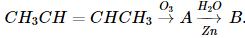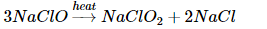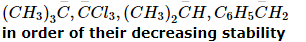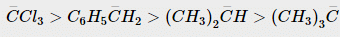# WBJEE Chemistry Test - 13

## 40 Questions MCQ Test WBJEE Sample Papers, Section Wise & Full Mock Tests | WBJEE Chemistry Test - 13

Description
Attempt WBJEE Chemistry Test - 13 | 40 questions in 60 minutes | Mock test for JEE preparation | Free important questions MCQ to study WBJEE Sample Papers, Section Wise & Full Mock Tests for JEE Exam | Download free PDF with solutions
QUESTION: 1

Solution:
QUESTION: 2

Solution:
QUESTION: 3

### The addition of HCN to carbonyl compounds is an example of

Solution:
QUESTION: 4
A bond formed between two elements having electronegativity 1.2 and 3.0 respectively is most likely
Solution:
QUESTION: 5
Dimethyl glyoxime in a suitable solvent was refluxed for 10 minutes with pure pieces of nickel sheet, it will result in
Solution:
QUESTION: 6
What happens when conc. H₂SO₄ is treated with sugar?
Solution:
QUESTION: 7
If the radius of first Bohr orbit be a₀, then the radius of the third orbit of hydrogen would be
Solution:
QUESTION: 8
Which of the following alkyl group has the maximum + I effect?
Solution:
QUESTION: 9
AgCl is soluble in
Solution: AgCl is a sparingly soluble salt and in its aqueous the following equilibrium exists-
AgCl Ag+ + Cl-
When NH4OH is added to a saturated solution of AgCl, Ag+ ions are removed from the system as soluble (Ag(NH3)2)+1 ions and the previous equilibrium is disturbed. To maintain the equlibrium constant more AgCl goes into solution. Therefore AgCl becomes more soluble in NH4OH solution
QUESTION: 10
The heat of neutralisation is determined by
Solution:
QUESTION: 11
According to Le-Chatelier's principle, adding heat to a solid and liquid in equilibrium will cause the
Solution:
QUESTION: 12
Which of these does not influence the rate of reaction?
Solution:
QUESTION: 13
All the naturally occurring processes proceed spontaneously in a direction which leads to
Solution:
QUESTION: 14
Alizarin belongs to the class of
Solution:
QUESTION: 15
A compound with molecular formula C₇H₁₆ shows optical isomerism , the compound will be
Solution:
QUESTION: 16

In which of the following complex ion, the central metal ion is in a state of sp3 d2  hybridisation?

Solution:
QUESTION: 17
Roasted ore is reduced to metal by
Solution:
QUESTION: 18

If 0.44 g of a colourless oxide of nitrogen occupies 224 ml at 1520 mm hg and 273oC, then the compound is

Solution:
QUESTION: 19
Which of the following processes does not involve a catalyst?
Solution:
QUESTION: 20
The metal used for making radiation shield is
Solution:
QUESTION: 21
The true statement for the acids of phosphorus H₃PO₂,H₃PO₃ and H₃PO₄ is
Solution:
QUESTION: 22
Which of the following metal evolves hydrogen on reacting with cold dilute HNO₃ ?
Solution:
QUESTION: 23
Which of the following will show geometrical isomerism?
Solution:
QUESTION: 24
Heaviest particle is
Solution:
QUESTION: 25
Which of the following is a strongest base?
Solution:
QUESTION: 26
The turbidity of a polymer solution measures
Solution:
QUESTION: 27
An example of a natural biopolymer is
Solution:
QUESTION: 28
Which of the following reactions involves oxidation and reduction?
Solution:
QUESTION: 29

How many Cl- ions are there around Na⁺ ion in NaCl crystal?

Solution:
QUESTION: 30
Consider the following statements about conformational isomers :
1. They are interconverted by rotation about single bond.
2. The energy barrier separating them is less than 15 kcal/mole.
3. They are best represented by means of Fischer Projection formulae.
Of these statements
Solution:
QUESTION: 31

In the following sequence of reactions, the alkene affords the compound 'B'The compound B is

Solution:
QUESTION: 32

Electrolysis of dilute aqueous NaCl solution was carried out by passing 10 milli ampere current. The time required to liberate 0.01 mol of H2 gas at the cathode is (1 Faraday = 96500 C mol-1)

Solution:

According to Faraday's first law of electrolysis:
m = EIt/F
Where:
m = mass of substance
E = equivalent weight of substance
I = current in amperes
t = time required
F = 96,500 CoulombsQUESTION: 33

The vapour pressure of water at 20oC is 17.5 mm Hg. If 18 g of glucose (C₆H₁₂O₆) is added to 178.2 g of water at 20oC , the vapour pressure of the resulting solution will be

Solution:
QUESTION: 34

Which of the following is correct about the reaction?Solution:
QUESTION: 35

Arrange the carbanions,Solution:

More the number of alkyl groups on the carbon atom carrying the negative charge, less stable is the carbanion. However, benzyl carbanions are stabilized by resonance. Further, the presence of electron withdrawing group (-Cl) increase the stability of carbanion.Thus, the order of their decreasing stability :QUESTION: 36

In the isothermal expansion of an ideal gas

Solution:
QUESTION: 37

Which of the following salt are insoluble in water?

Solution:
QUESTION: 38

Beryllium is the first member of group II and forms covalent compounds which are said to be electron deficient. In many ways, beryllium resembles aluminium. Which of the following are possible ?

Solution:
QUESTION: 39

The reagents , NH4Cl and aqueous NH3 will precipitate :

Solution:
QUESTION: 40

Statements that are true among the following are:

Solution:Use Code STAYHOME200 and get INR 200 additional OFF Use Coupon Code